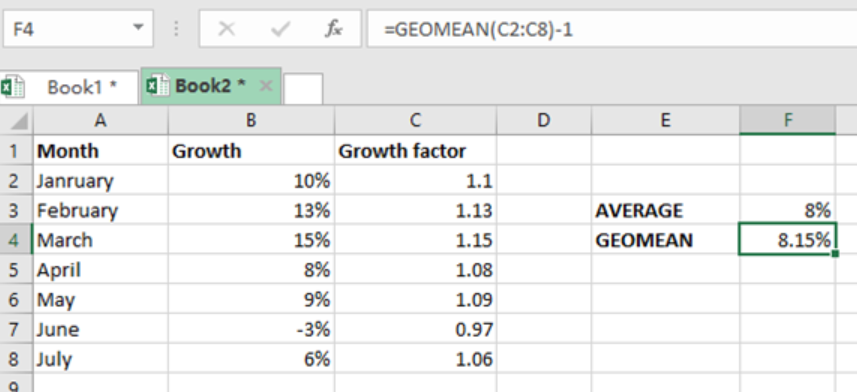Get instant live expert help with Excel or Google Sheets“My Excelchat expert helped me in less than 20 minutes, saving me what would have been 5 hours of work!”

#### Post your problem and you’ll get Expert help in seconds.

Your message must be at least 40 characters
Our professional Expert are available now. Your privacy is guaranteed.

# Excel GEOMEAN Function

If we want to find the geometric mean of an array of numbers, we can use the Excel GEOMEAN function. Note that this function can only work with positive. This article provides a clear guide with illustrative examples on how to use the Excel GEOMEAN function to calculate the geometric mean of numbers.Figure 1: Using GEOMEAN function

## Syntax of the formula

`=GEOMEAN (number1, [number2]….)`

Where;

• Number1- refers to the first value or reference
• Number2- refers to the second value or reference, it is optional.

## Explanation

GEOMEAN function in excel calculates the average rate of return of a set of values which is calculated using the products of the terms. In our example above, we have used the GEOMEAN function to calculate the monthly growth rate. This has been made possible by the use of the growth factor in column C, then we subtract 1.

The formula in cell F4 of our formula is;

`=GEOMEAN(C2:C8)-1`

## Things to remember

It is important to remember that arguments can be numbers, names, arrays or cell references which contain numbers.

Also, empty cells or cells that contain text or logical values are ignored by the GOMEAN function.

## Instant Connection to an Expert through our Excelchat Service

Most of the time, the problem you will need to solve will be more complex than a simple application of a formula or function. If you want to save hours of research and frustration, try our live Excelchat service! Our Excel Experts are available 24/7 to answer any Excel question you may have. We guarantee a connection within 30 seconds and a customized solution within 20 minutes.